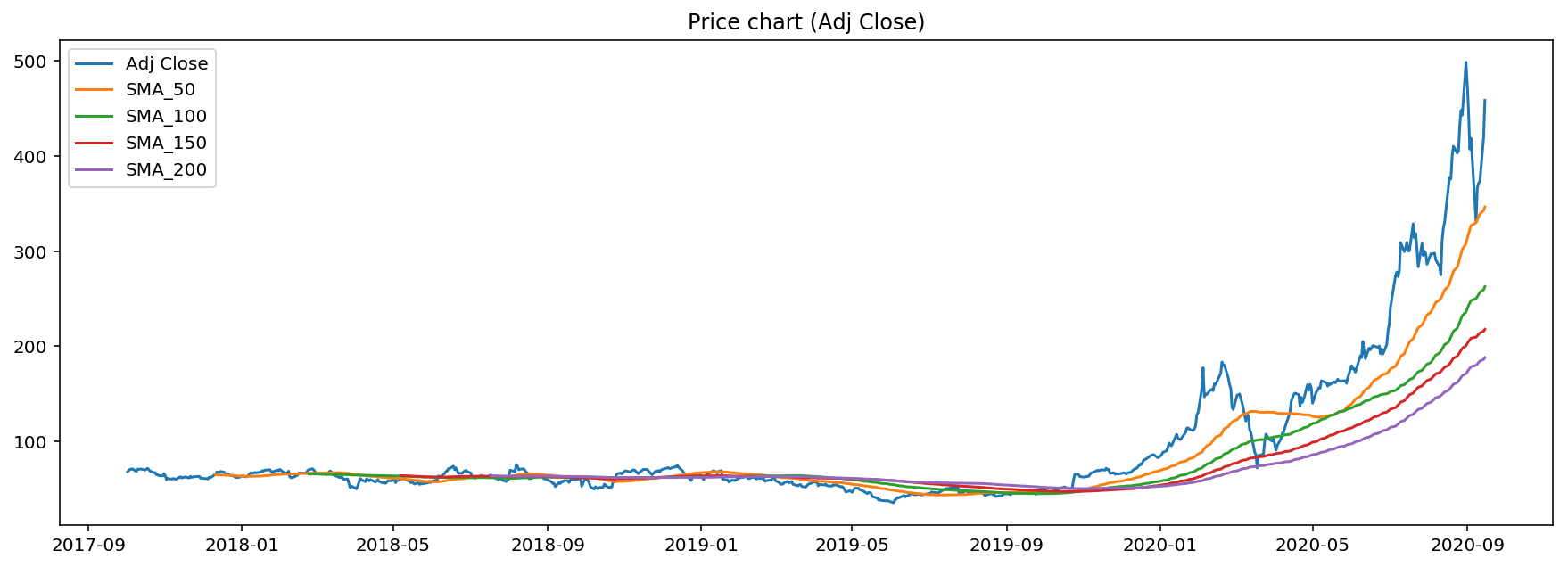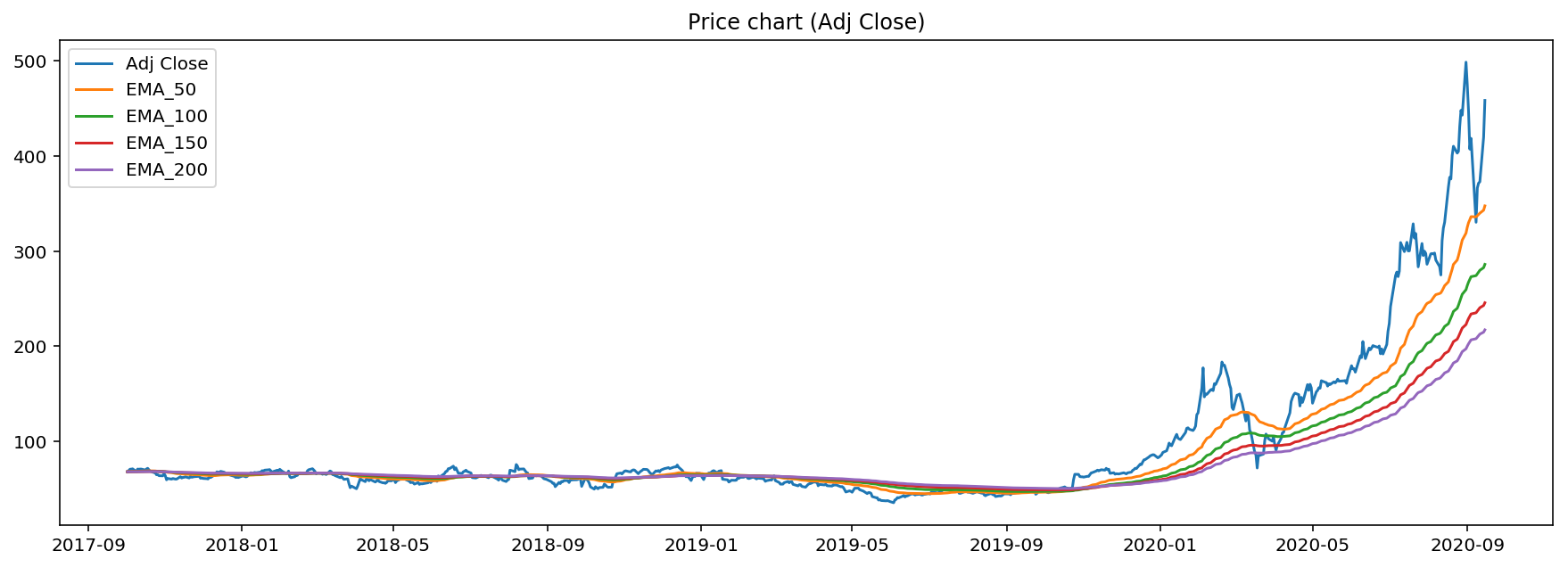## Simple and exponential moving averages for stocks in python¶

Moving averages (MAs) are basic, yet very powerful tool in technical analysis. Stock price typically finds support/resistance in vicinity of various significant moving averages.

Moving averages are especially useful when stock is in developed uptrend or downtrend.

We distinguish 2 types of MAs:

• simple moving average
• exponencial moving average

Simple moving average applies equal weight to all datapoints, on the other hand exponencial moving average gives more importance to recent price action.

In this article we will be computing both simple and exponencial moving averages for 50, 100, 150 and 200 point window.

Script is intended to be eventually part of a trading bot.

In :
from IPython.core.display import display, HTML
display(HTML("<style>.container { width:100% !important; }</style>"))
%matplotlib inline

from IPython.display import set_matplotlib_formats
set_matplotlib_formats('retina')

In :
#optional installations:

In :
import sys
import os

# ___library_import_statements___
import pandas as pd

# for pandas_datareader, otherwise it might have issues, sometimes there is some version mismatch
pd.core.common.is_list_like = pd.api.types.is_list_like

# make pandas to print dataframes nicely
pd.set_option('expand_frame_repr', False)

import numpy as np
import matplotlib.pyplot as plt
import datetime
import time

import yfinance as yahoo_finance

#                           ___variables___
# ------------------------------------------------------------------------------

#ticker = 'AAPL'
#ticker = 'SPY'
#ticker = 'QQQ'
ticker = 'TSLA'

start_time = datetime.datetime(2017, 10, 1)
#end_time = datetime.datetime(2019, 1, 20)
end_time = datetime.datetime.now().date().isoformat()     # today

#                       __function_definitions__
# ------------------------------------------------------------------------------

def get_data(ticker):
# yahoo gives only daily historical data
attempts = 0
connected = False
while not connected:
try:
ticker_df = web.get_data_yahoo(ticker, start=start_time, end=end_time)
connected = True
print('connected to yahoo')
except Exception as e:
print("type error: " + str(e))
time.sleep( 5 )
attempts += 1
if attempts >= 10:
connected = True
pass

# use numerical integer index instead of date
ticker_df = ticker_df.reset_index()

return ticker_df

In :
def computeSMA(data, window):
# simple moving average
sma = data.rolling(window=window).mean()

return sma

def computeEMA(data, span):
# simple moving average

return ema

def construct_df(ticker):
#get data from yahoo API
df = get_data(ticker)
# compute both types of moving averages
for i in range(50, 250, 50):
#print(i)
for i in range(50, 250, 50):
#print(i)

return df


In :
df = construct_df(ticker)

connected to yahoo

In :
df.tail()

Out:
Date High Low Open Close Volume Adj Close SMA_50 SMA_100 SMA_150 SMA_200 EMA_50 EMA_100 EMA_150 EMA_200
739 2020-09-09 369.000000 341.510010 356.600006 366.279999 79465800.0 366.279999 332.921317 252.166379 211.096652 181.417209 337.088805 276.021493 236.943588 209.569784
740 2020-09-10 398.989990 360.559998 386.209991 371.339996 84930600.0 371.339996 336.028877 254.387059 212.573639 182.940869 338.431989 277.908988 238.723673 211.179438
741 2020-09-11 382.500000 360.500000 381.940002 372.720001 60717500.0 372.720001 339.004757 256.740819 214.061012 184.468129 339.776617 279.786434 240.498458 212.786806
742 2020-09-14 420.000000 373.299988 380.950012 419.619995 82377400.0 419.619995 342.562517 259.472799 215.830106 186.237309 342.907730 282.555415 242.870929 214.844848
743 2020-09-15 461.940002 430.709991 436.559998 458.170013 80614387.0 458.170013 346.239597 262.643239 217.852066 188.196869 347.427819 286.032932 245.722573 217.265994
In :
def plot_data_SMA(df):
plt.figure(figsize=(15,5))

for i in range(50, 250, 50):
plt.plot(df['Date'], df['SMA_{}'.format(i)])

plt.legend(loc='best')
plt.show()

def plot_data_EMA(df):
plt.figure(figsize=(15,5))

for i in range(50, 250, 50):
plt.plot(df['Date'], df['EMA_{}'.format(i)])

plt.legend(loc='best')
plt.show()



### Simple moving average¶

In :
plot_data_SMA(df)### Exponencial moving average¶

In :
plot_data_EMA(df)From the plots we can see that Tesla (TSLA) stock found support pretty much exactly on 50 and 200 simple moving average.